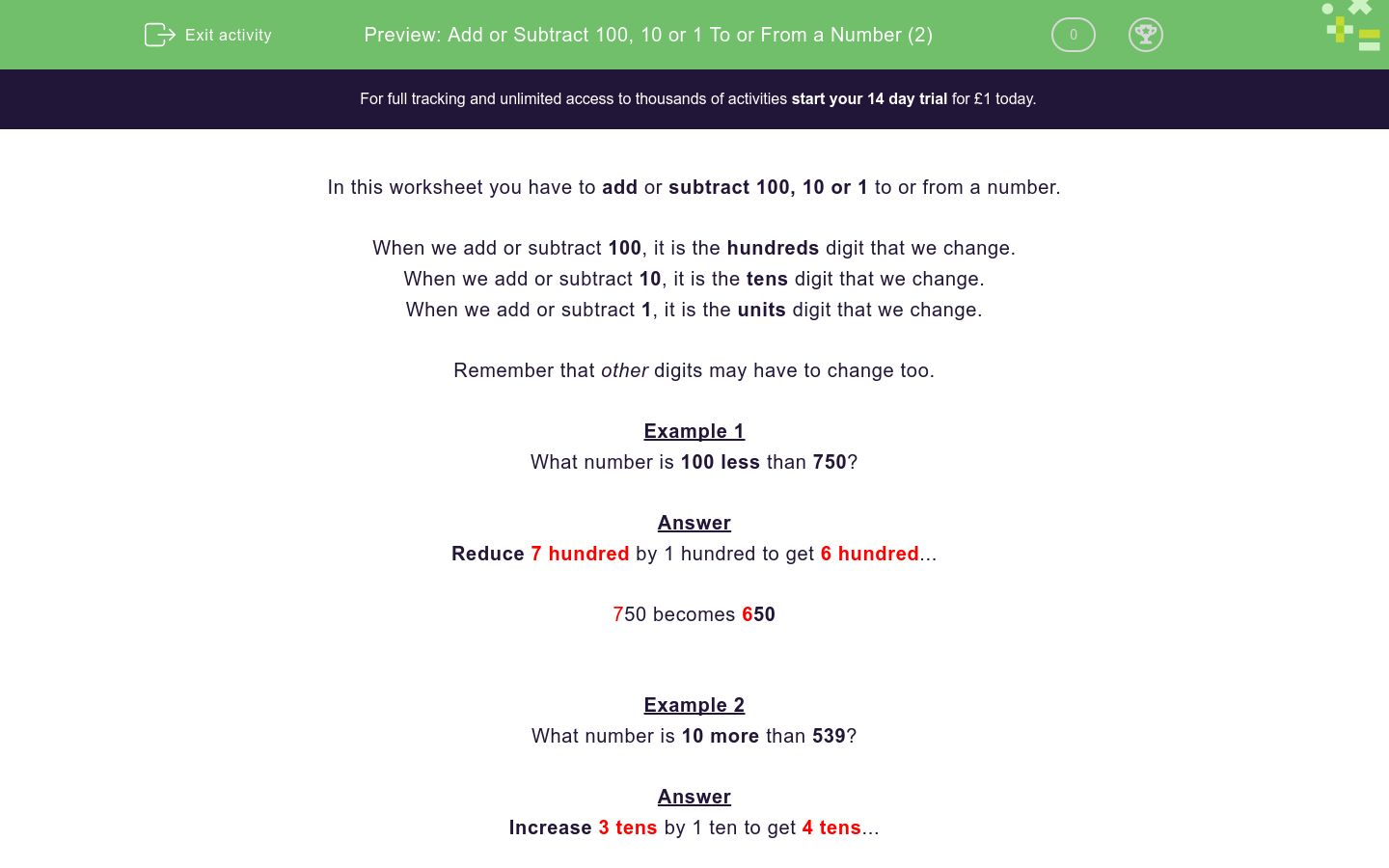# Add or Subtract 100, 10 or 1 To or From a Number (2)

In this worksheet, students add or subtract 100, 10 or 1 to or from a given number.Key stage:  KS 2

Curriculum topic:  Number: Number and Place Value

Curriculum subtopic:  Find 10 or 100 More or Less than a Given Number

Difficulty level:### QUESTION 1 of 10

In this worksheet you have to add or subtract 100, 10 or 1 to or from a number.

When we add or subtract 100, it is the hundreds digit that we change.

When we add or subtract 10, it is the tens digit that we change.

When we add or subtract 1, it is the units digit that we change.

Remember that other digits may have to change too.

Example 1

What number is 100 less than 750?

Reduce 7 hundred by 1 hundred to get 6 hundred...

750 becomes 650

Example 2

What number is 10 more than 539?

Increase 3 tens by 1 ten to get 4 tens...

539 becomes 549

Example 3

What number is 1 less than 539?

Reduce 9 units by 1 unit to get 8 units...

539 becomes 538

Example 4

What number is 10 less than 405?

Here we have to be careful, because we can't reduce the tens digit by 1.

So include the hundreds digit.

Think of it as reducing 40 tens by 1 ten to get 39 tens.

405 becomes 395

What number is 1 less than 945?

What number is 100 less than 909?

What number is 1 less than 219?

What number is 100 less than 465?

What number is 10 more than 473?

What number is 100 more than 663?

What number is 10 more than 887?

What number is 100 more than 323?

What number is 10 less than 402?

What number is 10 less than 887?

• Question 1

What number is 1 less than 945?

944
EDDIE SAYS
Reduce 5 units by 1 unit to get 4 units...
945 becomes 944
• Question 2

What number is 100 less than 909?

809
EDDIE SAYS
Reduce 9 hundred by 1 hundred to get 8 hundred...
909 becomes 809
• Question 3

What number is 1 less than 219?

218
EDDIE SAYS
Reduce 9 units by 1 unit to get 8 units...
219 becomes 218
• Question 4

What number is 100 less than 465?

365
EDDIE SAYS
Reduce 4 hundred by 1 hundred to get 3 hundred...
465 becomes 365
• Question 5

What number is 10 more than 473?

483
EDDIE SAYS
Increase 7 tens by 1 ten to get 8 tens...
473 becomes 483
• Question 6

What number is 100 more than 663?

763
EDDIE SAYS
Increase 6 hundred by 1 hundred to get 7 hundred...
663 becomes 763
• Question 7

What number is 10 more than 887?

897
EDDIE SAYS
Increase 8 tens by 1 ten to get 9 tens...
887 becomes 897
• Question 8

What number is 100 more than 323?

423
EDDIE SAYS
Increase 3 hundred by 1 hundred to get 4 hundred...
323 becomes 423
• Question 9

What number is 10 less than 402?

392
EDDIE SAYS
Reduce 40 tens by 1 ten to get 39 tens...
402 becomes 392
• Question 10

What number is 10 less than 887?

877
EDDIE SAYS
Reduce 8 tens by 1 ten to get 7 tens...
887 becomes 877
---- OR ----

Sign up for a £1 trial so you can track and measure your child's progress on this activity.

### What is EdPlace?

We're your National Curriculum aligned online education content provider helping each child succeed in English, maths and science from year 1 to GCSE. With an EdPlace account you’ll be able to track and measure progress, helping each child achieve their best. We build confidence and attainment by personalising each child’s learning at a level that suits them.

Get startedStart your £1 trial today.
Subscribe from £10/month.